# Addition of Three 3-digit numbers without Regrouping | Maths Grade 2

### Addition of Three 3-digit numbers without Regrouping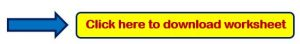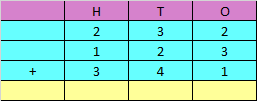Explanation:

Step 1: Adding the digits at ones place,

2 + 3 + 1 = 6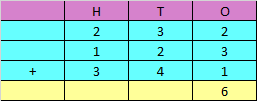Step 2: Adding the digits at tens place,

3 + 2 + 4 = 9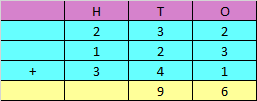Step 3: Adding the digits at hundreds place,

2 + 1 + 3 = 6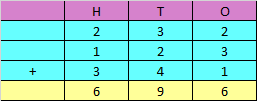Hence, the sum of the given numbers is 696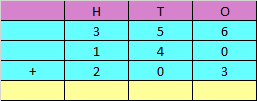Explanation:

Step 1: Adding the digits at ones place,

6 + 0 + 3 = 9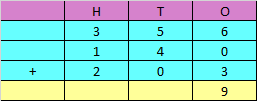Step 2: Adding the digits at tens place,

5 + 4 + 0 = 9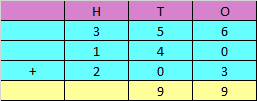Step 3: Adding the digits at hundreds place,

3 + 1 + 2 = 6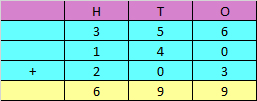Hence, the sum of the given numbers is 699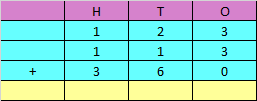Explanation:

Step 1: Adding the digits at ones place,

3 + 3 + 0 = 6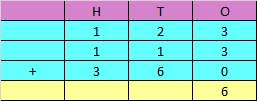Step 2: Adding the digits at tens place,

2 + 1 + 6 = 9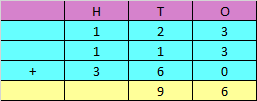Step 3: Adding the digits at hundreds place,

1 + 1 + 3 = 5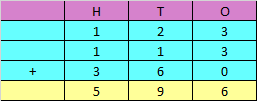Hence, the sum of the given numbers is 596